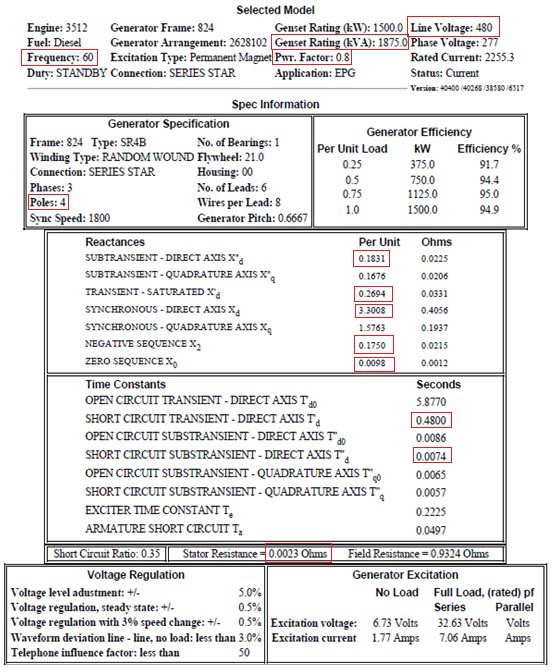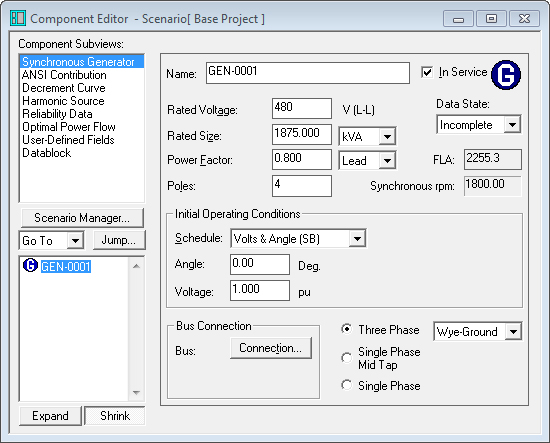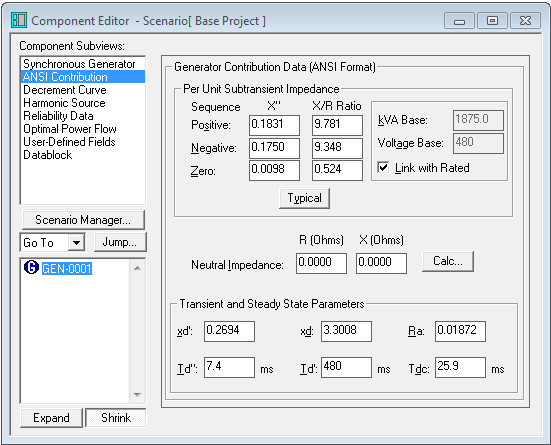Synchronous Generator Input Data

Answer: Make sure to use manufacturer data. Below is an example of generator input data from manufacturer.

Rated Voltage : 480 V

Rated Size : 1875 kVA

Power Factor : 0.8

Poles : 4

Zb = [(kVb) 2 x 1000]/ kVAb = [(0.48 kV) 2 x 1000]/ 1875kVA = 230.4kV2/1875kVA = 0.12288 ohms

Ra =0.0023 ohms ; Rapu = Ra/Zb = (0.0023 ohms)/ (0.12288 ohms) = s0.01872 pu

X"pos = 0.1831 pu X/Rpos = X"pos/ Rapu = 0.1831/0.01872 = 9.781

Xneg = 0.1750 pu X/Rneg = Xneg/ Rapu = 0.1750/0.01872 = 9.348

Xzero = 0.0098 pu X/Rzero = Xzero/ Rapu = 0.0098/0.01872 = 0.524

Xd' = 0.2694 pu

Xd = 3.3008 pu

Td" = 0.0074 seconds = 7.4 ms

Td' = 0.4800 seconds = 480 ms

Tdc = Xd" / (2pf * Ra) where Xd" = X"pos, f is frequency.

Tdc = 0.1831/ (2pi *60Hz * 0.01872) = 0.1831/ 7.061 = 0.0259 seconds = 25.9 ms